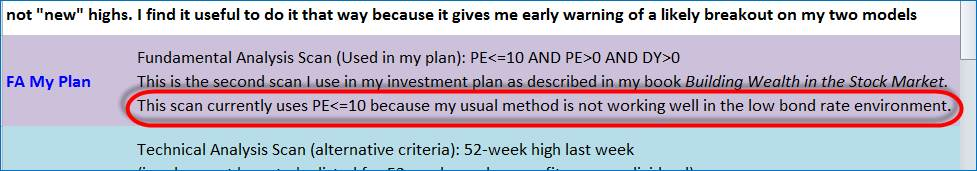In the current low interest rate environment (2015) are you still using 1.5% the bond rate to calculate the PE ratio for your fundamental analysis scans?

PE ratios are a rather crude, but commonly used measure of value. They work well in, say, real estate where the rental earnings stream is very predictable. However, the earnings stream of companies requires predicting the future and because the earnings will fluctuate in the future, the PE ratio measure is flawed.

That said, in the absence of more sophisticated analysis  it will keep investors out of trouble to some extent. So, a stock with a PE ratio of 10 might be cheap, one with a PE ratio of 15 may be fair value, ones with PE ratios of 25, 30, 35 and higher are overvalued and might be experiencing very high growth (which will not continue – nothing grows to the sky).

Benjamin Graham suggested buying large long established companies with a PE ratio of 15 or less, acknowledging that they are often hard to find, because they will be good businesses and usually have higher PE ratios than this.

Now enter the problem of interest rates (we talk of bond rates because 10-year government bonds are seen as risk free investments).

If we are to value a business, in principle we should estimate all future earnings (or dividends) and discount that stream of earnings back to current value. That is what the business is worth. To do that we have to select a discount rate. The bond rate is often used, but higher or lower rates might be chosen in various circumstances. The problem is that bond rates can swing up or down quite a lot and this changes quite dramatically the estimated current value of the business.

So the valuation method is easy in principle, but two things have to be estimated or assumed – future earnings and the discount rate. Depending on our earnings estimate and the discount rate we choose, the value can vary very greatly.

My use of the bond rate/PE ratio calculation is somewhat different in that it tries to come to terms with what PE ratio is reasonable value. It uses the current price and current earnings, dodging the valuation/discount rate issue in part (in part because it assumes earnings will continue at the current level).

This is explained in Building Wealth in the Stock Market, where I explained the logic. Bonds are risk free investments. Stocks are risky. Therefore, we should demand a higher return from stocks – what we call a premium for risk. If the bond rate never varied much, this would be easy – we might say we want a 50% risk premium – hence if the bond rate was 6%, we calculate the fair value PE ratio as  100/(6*1.5) = 11.1x.

50% was a big risk premium, so it gave a PE ratio that was indicating undervalued stocks and that was useful for my fundamental analysis filter.

That was fine when the bond rate was in a “normal” range. Fair value longer term seems to be a PE ratio of 12x to 16x, so 11x was below fair value.

However, we have for some time now been in a very low bond rate environment. Recently the bond rate fell to 2.55%, so the calculation became 100/(2.55*1.5)= 26x. This would be absurdly high.

As you know from the weekly market scans, I abandoned that method for the fundamental analysis scan a couple of years ago and have been using an arbitrary PE ratio of 10:The fundamental analysis scan is not my major scan, but I use it some of the time looking for undervalued stocks. Most stocks with low PE ratios are in trouble. My main scan is the technical analysis scan, which picks up stocks that fit my two models.

These scans are only a way to sift the 2,100+ securities available to a small list of stocks for further analysis. Estimating value is a later step as you know from my stock investment journals.

Keyword: